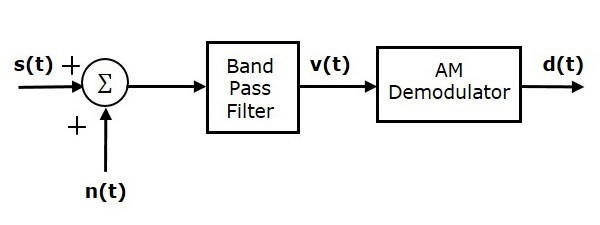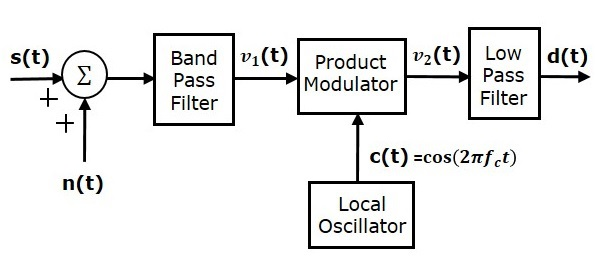# Analog Communication - SNR Calculations

In this chapter, let us calculate Signal to Noise Ratios and Figure of Merits of various modulated waves, which are demodulated at the receiver.

## Signal to Noise Ratio

Signal-to-Noise Ratio (SNR) is the ratio of the signal power to noise power. The higher the value of SNR, the greater will be the quality of the received output.

Signal-to-Noise Ratio at different points can be calculated using the following formulas.

Input SNR = $\left ( SNR \right )_I= \frac{Average \:\: power \:\:of \:\:modulating \:\:signal}{Average\:\: power \:\:of \:\:noise \:\:at \:\:input}$

Output SNR = $\left ( SNR \right )_O= \frac{Average \:\: power \:\:of \:\:demodulated \:\:signal}{Average\:\: power \:\:of \:\:noise \:\:at \:\:output}$

Channel SNR = $\left ( SNR \right )_C= \frac{Average \:\: power \:\:of \:\:modulated \:\:signal}{Average\:\: power \:\:of \:\:noise \:\:in \:\:message \:\:bandwidth}$

## Figure of Merit

The ratio of output SNR and input SNR can be termed as Figure of Merit. It is denoted by F. It describes the performance of a device.

$$F=\frac {\left ( SNR \right )_O}{\left ( SNR \right )_I}$$

Figure of merit of a receiver is

$$F=\frac {\left ( SNR \right )_O}{\left ( SNR \right )_C}$$

It is so because for a receiver, the channel is the input.

## SNR Calculations in AM System

Consider the following receiver model of AM system to analyze noise.We know that the Amplitude Modulated (AM) wave is

$$s\left ( t \right )=A_c\left [ 1+k_am\left ( t \right ) \right ] \cos\left ( 2 \pi f_ct \right )$$

$$\Rightarrow s\left ( t \right )=A_c \cos \left ( 2 \pi f_ct \right )+A_ck_am\left ( t \right ) \cos\left ( 2 \pi f_ct \right )$$

Average power of AM wave is

$$P_s=\left ( \frac{A_c}{\sqrt{2}} \right )^2+\left ( \frac{A_ck_am\left ( t \right )}{\sqrt{2}} \right )^2=\frac{{A_{c}}^{2}}{2}+\frac{{A_{c}}^{2}{k_{a}}^{2}P}{2}$$

$$\Rightarrow P_s=\frac{{A_{c}}^{2}\left ( 1+{k_{a}}^{2}P \right )}{2}$$

Average power of noise in the message bandwidth is

$$P_{nc}=WN_0$$

Substitute, these values in channel SNR formula

$$\left ( SNR \right )_{C,AM}=\frac{Average \:\: Power \:\: of \:\: AM \:\: Wave}{Average \:\: Power \:\: of \:\: noise \:\: in \:\: message \:\: bandwidth}$$

$$\Rightarrow \left ( SNR \right )_{C,AM}=\frac{{A_{c}}^{2}\left ( 1+ {k_{a}}^{2}\right )P}{2WN_0}$$

Where,

• P is the power of the message signal=$\frac{{A_{m}}^{2}}{2}$

• W is the message bandwidth

Assume the band pass noise is mixed with AM wave in the channel as shown in the above figure. This combination is applied at the input of AM demodulator. Hence, the input of AM demodulator is.

$$v\left ( t \right )=s\left ( t \right )+n\left ( t \right )$$

$\Rightarrow v\left ( t \right )=A_c\left [ 1+k_am\left ( t \right ) \right ] \cos\left ( 2 \pi f_ct \right )+$

$\left [ n_1\left ( t \right ) \cos\left ( 2 \pi f_ct \right ) - n_Q\left ( t \right ) \sin \left ( 2 \pi f_ct \right )\right ]$

$\Rightarrow v\left ( t \right )=\left [ A_c+A_ck_am\left ( t \right )+n_1\left ( t \right ) \right ] \cos\left ( 2 \pi f_ct \right )-n_Q\left ( t \right ) \sin\left ( 2 \pi f_ct \right )$

Where $n_I \left ( t \right )$ and $n_Q \left ( t \right )$ are in phase and quadrature phase components of noise.

The output of AM demodulator is nothing but the envelope of the above signal.

$$d\left ( t \right )=\sqrt{\left [ A_c+A_cK_am\left ( t \right )+n_I\left ( t \right ) \right ]^2+\left ( n_Q\left ( t \right ) \right )^2}$$

$$\Rightarrow d\left ( t \right )\approx A_c+A_ck_am\left ( t \right )+n_1\left ( t \right )$$

Average power of the demodulated signal is

$$P_m=\left ( \frac{A_ck_am\left ( t \right )}{\sqrt{2}} \right )^2=\frac{{A_{c}}^{2}{k_{a}}^{2}P}{2}$$

Average power of noise at the output is

$$P_no=WN_0$$

Substitute, these values in output SNR formula.

$$\left ( SNR \right )_{O,AM}= \frac {Average \:\: Power \:\: of \:\: demodulated \:\: signal }{Average \:\: Power \:\: of \:\: noise \:\: at \:\: Output}$$

$$\Rightarrow \left ( SNR \right )_{O,AM}=\frac{{A_{c}}^{2}{k_{a}}^{2}P}{2WN_0}$$

Substitute, the values in Figure of merit of AM receiver formula.

$$F=\frac{\left ( SNR \right )_{O,AM}}{\left ( SNR \right )_{C,AM}}$$

$$\Rightarrow F=\left ( \frac{{A_{c}^{2}}{k_{a}^{2}}P}{2WN_0} \right )/\left ( \frac{{A_{c}}^{2}\left ( 1+ {k_{a}}^{2}\right )P}{2WN_0} \right )$$

$$\Rightarrow F=\frac{{K_{a}}^{2}P}{1+{K_{a}}^{2}P}$$

Therefore, the Figure of merit of AM receiver is less than one.

## SNR Calculations in DSBSC System

Consider the following receiver model of DSBSC system to analyze noise.We know that the DSBSC modulated wave is

$$s\left ( t \right )=A_cm\left ( t \right ) \cos\left ( 2 \pi f_ct \right )$$

Average power of DSBSC modulated wave is

$$P_s=\left ( \frac{A_cm\left ( t \right )}{\sqrt{2}} \right )^2=\frac{{A_{c}}^{2}P}{2}$$

Average power of noise in the message bandwidth is

$$P_{nc}=WN_0$$

Substitute, these values in channel SNR formula.

$$\left ( SNR \right )_{C,DSBSC}=\frac{Average \:\: Power \:\: of \:\: DSBSC \:\: modulated \:\: wave}{Average \:\: Power \:\: of \:\: noise \:\: in \:\: message \:\: bandwidth}$$

$$\Rightarrow \left ( SNR \right )_{C,DSBSC}=\frac{{A_{c}}^{2}P}{2WN_0}$$

Assume the band pass noise is mixed with DSBSC modulated wave in the channel as shown in the above figure. This combination is applied as one of the input to the product modulator. Hence, the input of this product modulator is

$$v_1\left ( t \right )=s\left ( t \right )+n\left ( t \right )$$

$$\Rightarrow v_1\left ( t \right )=A_cm\left ( t \right ) \cos \left ( 2 \pi f_ct \right )+\left [ n_I\left ( t \right ) \cos\left ( 2 \pi f_ct \right ) - n_Q\left ( t \right ) \sin \left ( 2 \pi f_ct \right )\right ]$$

$$\Rightarrow v_1\left ( t \right )=\left [ A_cm \left ( t \right ) +n_I\left ( t \right ) \right ] \cos\left ( 2 \pi f_ct \right )-n_Q\left ( t \right ) \sin\left ( 2 \pi f_ct \right )$$

Local oscillator generates the carrier signal $c\left ( t \right )= \cos\left ( 2 \pi f_ct \right )$. This signal is applied as another input to the product modulator. Therefore, the product modulator produces an output, which is the product of $v_1\left ( t \right )$ and $c\left ( t \right )$.

$$v_2\left ( t \right )= v_1\left ( t \right )c\left ( t \right )$$

Substitute, $v_1\left ( t \right )$ and $c\left ( t \right )$ values in the above equation.

$$\Rightarrow v_2\left ( t \right )=\left ( \left [ A_cm\left ( t \right ) + n_I\left ( t \right )\right ] \cos\left ( 2 \pi f_ct \right )- n_Q\left ( t \right ) \sin\left ( 2 \pi f_ct \right ) \right ) \cos\left ( 2 \pi f_ct \right )$$

$$\Rightarrow v_2\left ( t \right )=\left [ A_c m\left ( t \right )+n_I\left ( t \right ) \right ] \cos^2\left ( 2 \pi f_ct \right )-n_Q\left ( t \right ) \sin\left ( 2 \pi f_ct \right ) \cos\left ( 2 \pi f_ct \right )$$

$$\Rightarrow v_2\left ( t \right )=\left [ A_c m\left ( t \right )+n_I\left ( t \right ) \right ] \left ( \frac{1+ \cos\left ( 4 \pi f_ct \right )}{2} \right ) -n_Q\left ( t \right )\frac{ \sin\left ( 4 \pi f_ct \right )}{2}$$

When the above signal is applied as an input to low pass filter, we will get the output of low pass filter as

$$d\left ( t \right )=\frac{\left [ A_c m\left ( t \right )+n_I\left ( t \right ) \right ]}{2}$$

Average power of the demodulated signal is

$$P_m=\left ( \frac{A_cm\left ( t \right )}{2\sqrt{2}} \right )^2=\frac{{A_{c}}^{2}P}{8}$$

Average power of noise at the output is

$$P_{no}=\frac{WN_0}{4}$$

Substitute, these values in output SNR formula.

$$\left ( SNR \right )_{O,DSBSC}= \frac {Average \:\: Power \:\: of \:\: demodulated \:\: signal }{Average \:\: Power \:\: of \:\: noise \:\: at \:\: Output}$$

$$\Rightarrow \left ( SNR \right )_{O,DSBSC}=\left ( \frac{{A_{c}}^{2}P}{8} \right )/ \left ( \frac{WN_0}{4} \right )=\frac{{A_{c}}^{2}P}{2WN_0}$$

Substitute, the values in Figure of merit of DSBSC receiver formula.

$$F=\frac{\left ( SNR \right )_{O,DSBSC}}{\left ( SNR \right )_{C,DSBSC}}$$

$$\Rightarrow F= \left ( \frac{{A_{c}}^{2}P}{2WN_0} \right )/ \left ( \frac{{A_{c}}^{2}P}{2WN_0} \right )$$

$$\Rightarrow F= 1$$

Therefore, the Figure of merit of DSBSC receiver is 1.

## SNR Calculations in SSBSC System

Consider the following receiver model of SSBSC system to analyze noise.We know that the SSBSC modulated wave having lower sideband is

$$s\left ( t \right )=\frac{A_mA_c}{2} \cos \left [ 2 \pi\left ( f_c-f_m \right )t \right ]$$

Average power of SSBSC modulated wave is

$$P_s=\left ( \frac{A_mA_c}{2\sqrt{2}} \right )^2=\frac{{A_{m}}^{2}{A_{c}}^{2}}{8}$$

Average power of noise in the message bandwidth is

$$P_{nc}=WN_0$$

Substitute, these values in channel SNR formula.

$$\left ( SNR \right )_{C,SSBSC}= \frac {Average \:\: Power \:\: of \:\: SSBSC \:\: modulated \:\: wave}{Average \:\: Power \:\: of \:\: noise \:\: in\:\: message \:\: bandwidth}$$

$$\Rightarrow \left ( SNR \right )_{C,SSBSC}=\frac{{A_{m}}^{2}{A_{c}}^{2}}{8WN_0}$$

Assume the band pass noise is mixed with SSBSC modulated wave in the channel as shown in the above figure. This combination is applied as one of the input to the product modulator. Hence, the input of this product modulator is

$$v_1\left ( t \right )=s\left ( t \right )+n\left ( t \right )$$

$$v_1\left ( t \right )=\frac{A_mA_c}{2} \cos\left [ 2 \pi \left ( f_c-f_m \right )t \right ] + n_I\left ( t \right ) \cos\left ( 2 \pi f_ct \right )-n_Q\left ( t \right ) \sin \left ( 2 \pi f_ct \right )$$

The local oscillator generates the carrier signal $c\left ( t \right )= \cos \left ( 2 \pi f_ct \right )$. This signal is applied as another input to the product modulator. Therefore, the product modulator produces an output, which is the product of $v_1\left ( t \right )$ and $c\left ( t \right )$.

$$v_2\left ( t \right )=v_1\left ( t \right )c \left ( t \right )$$

Substitute, $v_1\left ( t \right )$ and $c\left ( t \right )$ values in the above equation.

$\Rightarrow v_2(t)= (\frac{A_mA_c}{2} \cos[ 2 \pi ( f_c-f_m )t ] + n_I ( t ) \cos ( 2 \pi f_ct )-$

$n_Q( t ) \sin ( 2 \pi f_ct ) )\cos ( 2 \pi f_ct )$

$\Rightarrow v_2\left ( t \right )=\frac{A_mA_c}{2} \cos\left [ 2 \pi \left ( f_c-f_m \right )t \right ] \cos\left ( 2 \pi f_ct \right )+$

$n_I\left ( t \right ) \cos^2\left ( 2 \pi f_ct \right )-n_Q\left ( t \right ) \sin\left ( 2 \pi f_ct \right ) \cos\left ( 2 \pi f_ct \right )$

$\Rightarrow v_2\left ( t \right )=\frac{A_mA_c}{4} \left \{ \cos\left [ 2 \pi\left ( 2f_c-f_m \right )t \right ] + \cos \left ( 2 \pi f_mt \right )\right \}+$

$n_I\left ( t \right )\left ( \frac{1+ \cos\left ( 4 \pi f_ct \right )}{2} \right )- n_Q\left ( t \right )\frac{\sin \left ( 4 \pi f_ct \right )}{2}$

When the above signal is applied as an input to low pass filter, we will get the output of low pass filter as

$$d\left ( t \right )=\frac{A_mA_c}{2} \cos\left ( 2 \pi f_mt \right )+\frac{n_I\left ( t \right )}{2}$$

Average power of the demodulated signal is

$$P_m=\left ( \frac{A_mA_c}{4\sqrt{2}} \right )^2=\frac{{A_{m}}^{2}{A_{c}}^{2}}{32}$$

Average power of noise at the output is

$$P_{no}=\frac{WN_0}{4}$$

Substitute, these values in output SNR formula

$$\left ( SNR \right )_{O,SSBSC}= \frac {Average \:\: Power \:\: of \:\: demodulated \:\: signal}{Average \:\: Power \:\: of \:\: noise \:\: at \:\: output}$$

$$\Rightarrow \left ( SNR \right )_{O,SSBSC}= \left ( \frac{{A_{m}}^{2}{A_{c}}^{2}}{32} \right )/\left ( \frac{WN_0}{4} \right )=\frac{{A_{m}}^{2}{A_{c}}^{2}}{8WN_0}$$

Substitute, the values in Figure of merit of SSBSC receiver formula

$$F=\frac{\left ( SNR \right )_{O,SSBSC}}{\left ( SNR \right )_{C,SSBSC}}$$

$$F=\left ( \frac{{A_{m}}^{2}{A_{c}}^{2}}{8WN_0} \right )/\left ( \frac{{A_{m}}^{2}{A_{c}}^{2}}{8WN_0} \right )$$

$$F=1$$

Therefore, the Figure of merit of SSBSC receiver is 1.